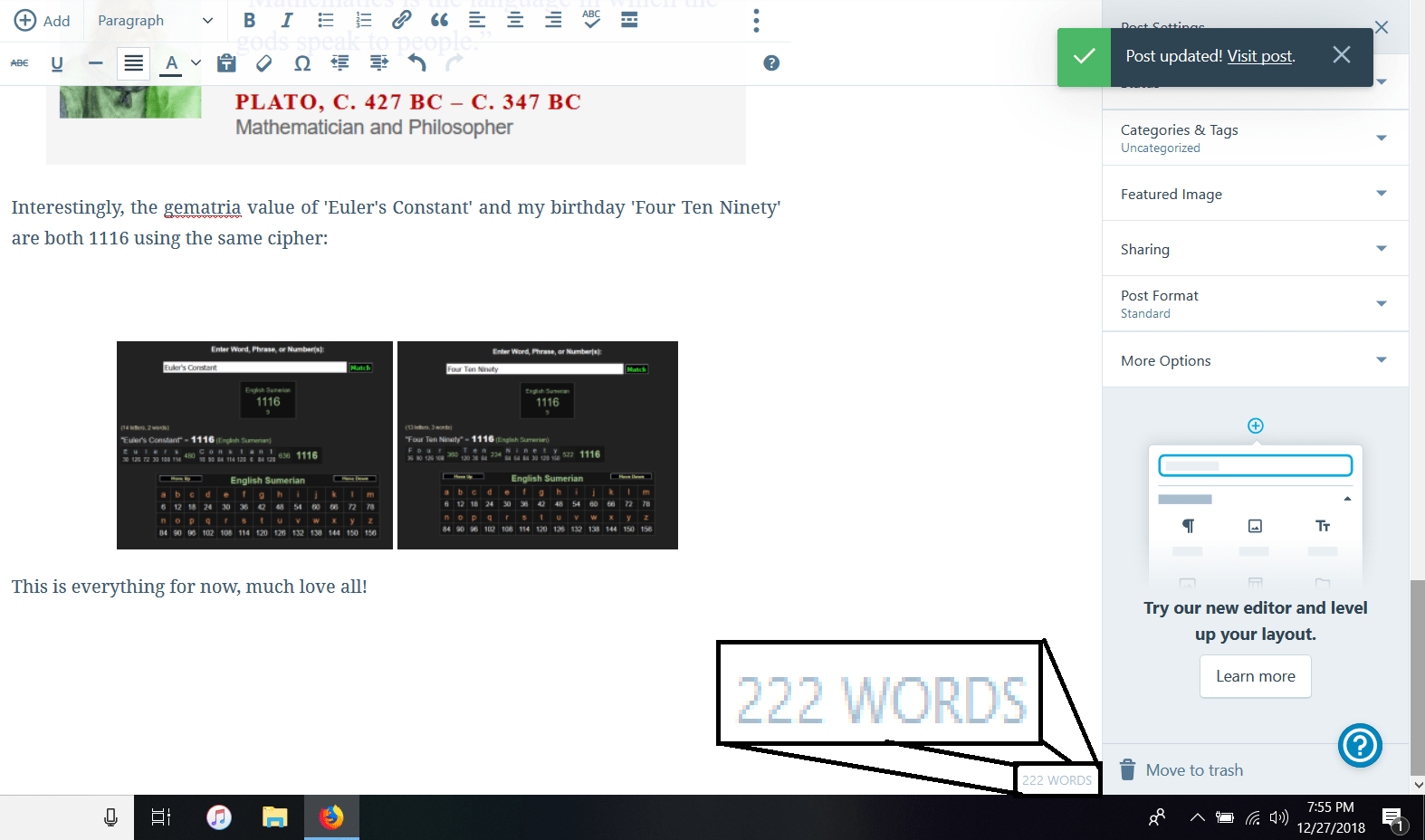## More Big Mathematical Synchronicities

So after watching some videos on gematria talking about various values related to Jesus the mathematical constant called ‘e’ or ‘Euler’s number’ came up, named after Leonhard Euler is a number similar to Pi, where it is referred to as one of the most important mathematical constants known today.

I am definitely not a mathematician and couldn’t explain it very well but it has to do with growth and compound interest:

https://en.wikipedia.org/wiki/E_(mathematical_constant)

Anyways this number is 2.71828 and goes on forever but it can be shortened just like Pi. And I thought okay I wonder what would happen if I played with this number and sure enough I got a hit.

If we plug in the magic number 410 and multiply it by 2.71 we get 1111.1: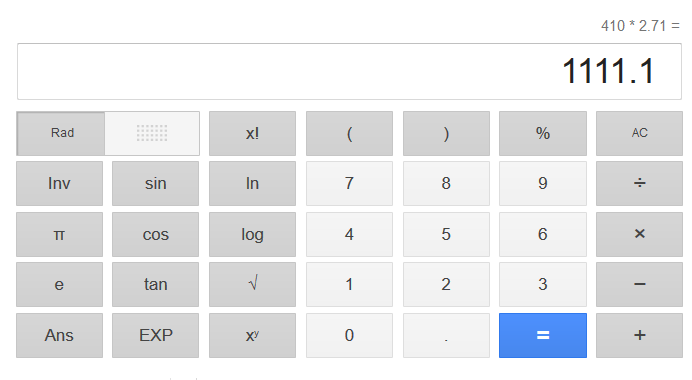So here we see yet again another expression of the number one related to the number 410. I would reiterate here that 410 divided by 369 is 1.11111 which goes on forever: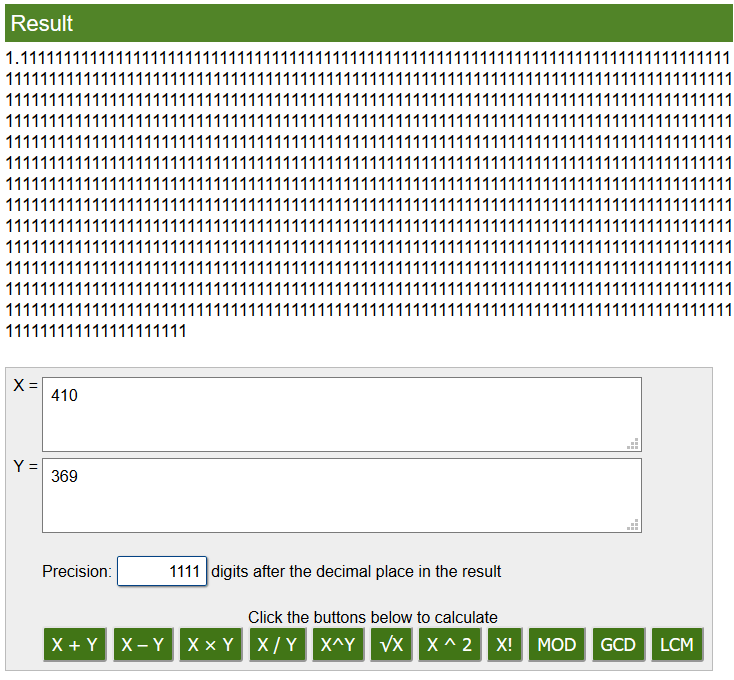So here we have more proof of 410 being a magic number that is connected to so many things, especially the most well-known mathematical equations. As I have discovered and as many have been saying for thousands of years, God/Source is math: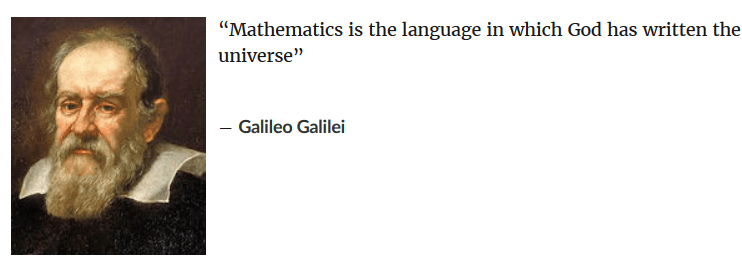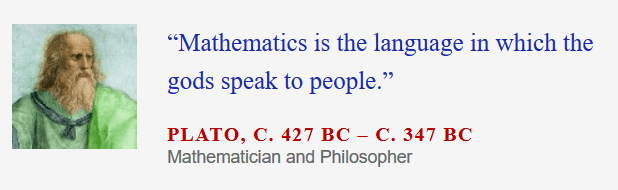Interestingly, the gematria value of ‘Euler’s Number’ and my birthday ‘Four Ten Ninety’ are both 1116 using the same cipher:

This is everything for now, much love all!